Custom SearchRADICALS To differentiate a function containing a radical, replace the radical by a fractional exponent; then find the derivative by apŁplying the appropriate theorems. EXAMPLE. Find the derivative of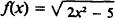SOLUTION: Replace the radical by the proper fractional exŁponent, such that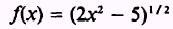and by Theorem 6EXAMPLE: Find the derivative of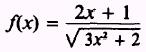SOL UTION: Replace the radical by the proper fractional exŁponent, thusAt this point a decision is in order. This problem may be solved by either writingand applying Theorem 6 in the denominator and then applying Theorem 5 for the quotient or writing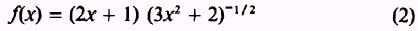and applying Theorem 6 for the second factor and then applying Theorem 4 for the product. The two methods of solution are completed individually as follows: Use equation (1):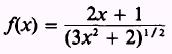Find the derivative of the denominator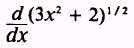by applying the power theorem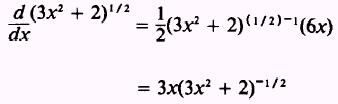The derivative of the numerator isNow apply Theorem 5:Multiply both numerator and denominator by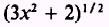and simplify:To find the same solution by a different method, use equaŁtion (2):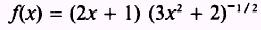Find the derivative of each factor: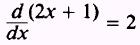andNow apply Theorem 4: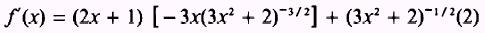Multiply both numerator and denominator by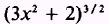such that,which agrees with the solution of the first method used. PRACTICE PROBLEMS: Find the derivatives of the following:ANSWERS: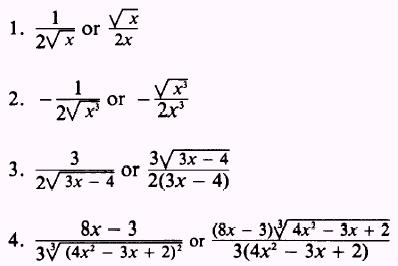Privacy Statement - Copyright Information. - Contact Us